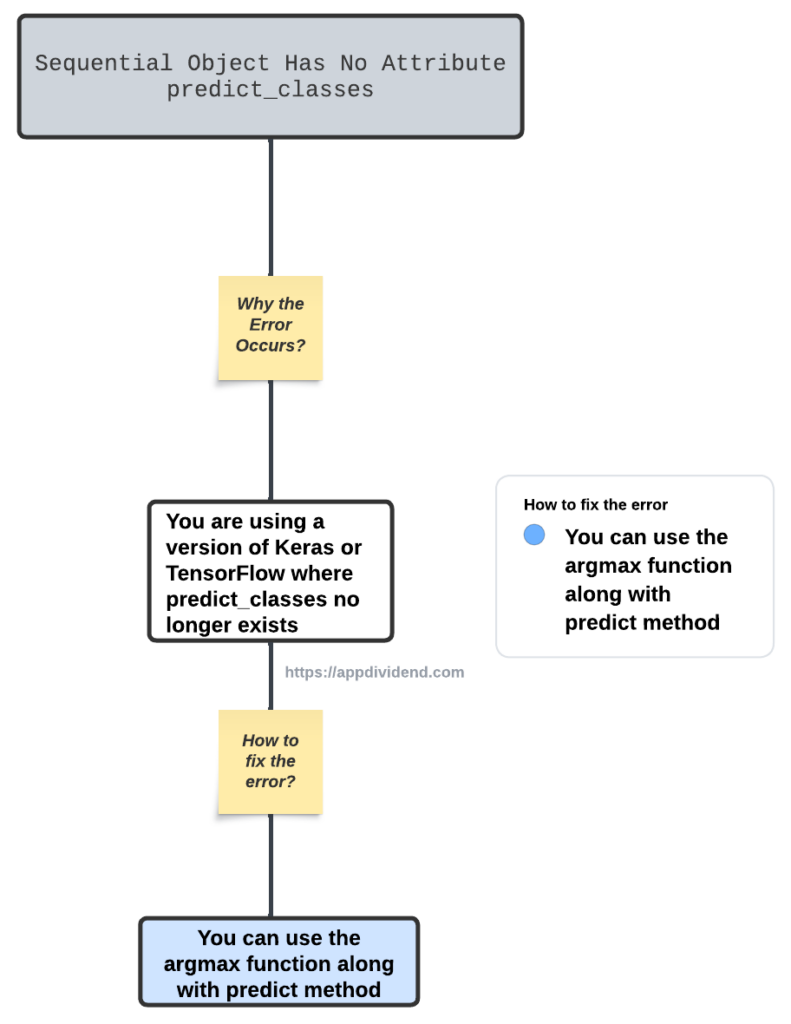# How to Fix AttributeError: ‘Sequential’ Object Has No Attribute ‘predict_classes’Diagram

AttributeError: ‘Sequential’ Object Has No Attribute ‘predict_classes’ error occurs because “you are using a version of Keras or TensorFlow where ‘predict_classes’ no longer exists.” In Keras, the ‘predict_classes’ was deprecated in Keras 2.3.0 and completely removed in Keras 2.4.0.

## Reproducing the error

``````from keras.models import Sequential

model = Sequential()

y_pred = model.predict_classes(validation_data)``````

Output

``````AttributeError: 'Sequential' object has no attribute 'predict_classes'
``````

## How to Fix it?

To fix the AttributeError: ‘Sequential’ Object Has No Attribute ‘predict_classes’, you can use the “argmax” function from the numpy library along with the “predict” method from your “Sequential” object. This combination will provide you with the same output as ‘predict_classes’.

``````import numpy as np
from tensorflow.keras.models import Sequential
from tensorflow.keras.layers import Dense

# Define the model
model = Sequential()

# Compile the model
metrics=['accuracy'])

# Assume 'data' is your input for prediction
data = np.random.random((500, 100))

# Use the 'predict' method to get the class probabilities
probabilities = model.predict(data)

# Use numpy's 'argmax' function to get the class predictions
class_predictions = np.argmax(probabilities, axis=-1)
``````

Output

``16/16 [==============================] - 0s 279us/step``

After running the above code in my console, you can see that the error is successfully fixed!